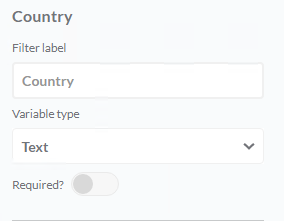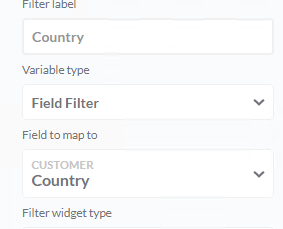# 'IN' operator taking a list of arguments

The following SQL syntax is a valid one.

``````SELECT
a.state,
sum(b.num_cars) * 10000.0/sum(a.data_2015) as proportion
FROM population as a
INNER JOIN "all" AS b
ON
a.state = b.state
WHERE a.state IN ('CA', 'TX', 'AZ')
GROUP BY
a.state
ORDER BY
proportion ASC
``````

How can I achieve the following?

``````SELECT
a.state,
sum(b.num_cars) * 10000.0/sum(a.data_2015) as proportion
FROM population as a
INNER JOIN "all" AS b
ON
a.state = b.state
[[ WHERE a.state IN ({{state}}) ]]
GROUP BY
a.state
ORDER BY
proportion ASC
``````

The focus is on the optional WHERE statement that should take a list of states (arguments).

Thank you.

Nope, doesn’t work. I had the same problem. I’ve now changed my dashboards from custom SQL to using views.
Views also make code reuse easier. If you’re not able to create views in your database due to security, take a look at:

@miya to achieve a similar result, you just need to add a normal field filter to your question. The lastest versions of Metabase already offer multiple selection filter.

``````SELECT
a.state,
sum(b.num_cars) * 10000.0/sum(a.data_2015) as proportion
FROM population as a
INNER JOIN "all" AS b
ON
a.state = b.state
[[ WHERE a.state IN ({{state}}) ]]
GROUP BY
a.state
ORDER BY
proportion ASC
``````

just use

``````SELECT
a.state,
sum(b.num_cars) * 10000.0/sum(a.data_2015) as proportion
FROM population as a
INNER JOIN "all" AS b
ON
a.state = b.state
WHERE 1=1
[[ AND ({{state}}) ]]
GROUP BY
a.state
ORDER BY
proportion ASC
``````
2 Likes

I’ve just realised where I’ve been going wrong! I’ve been using:when I should have been using:First option is just a straight text substitution whereas the 2nd does the correct SQL.

1 Like

The “special sauce” in the example above seems to be () surrounding the double {}. Otherwise there is no difference compared to similar examples.

The syntax in the quote above behaves as expected, similar to SQL “IN”, when linked by a field filter to an integer column, to process a default value of a space separated sequence of alphanumeric values such as:

123 456 789

However, when linked by a field filter to a varchar column, it doesn’t behave the same with default value of a space separated sequence such as:

123456789 192837465 918273645 987654321

Any ideas how to get past that?